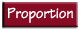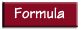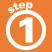Home    |    Teacher    |    Parents    |    Glossary    |    About UsA rate is a ratio that compares two different kinds of numbers, such as miles per hour or dollars per pound. A unit rate compares a quantity to its unit of measure. A unit price is a rate comparing the price of an item to its unit of measure.

The rate "miles per hour" gives distance traveled per unit of time. Problems using this type of rate can be solved using a proportion, or a formula.

On a vacation, Linda's family traveled 495 miles at 55 mph. How long did the trip take?Homework Help | Pre-Algebra | Ratios and ProportionsEmail this page to a friendSearch·  Ratios·  Proportions·  Distance, rate     and time·  Similar figuresFirst Glance In Depth Examples WorkoutDistance, rate and time